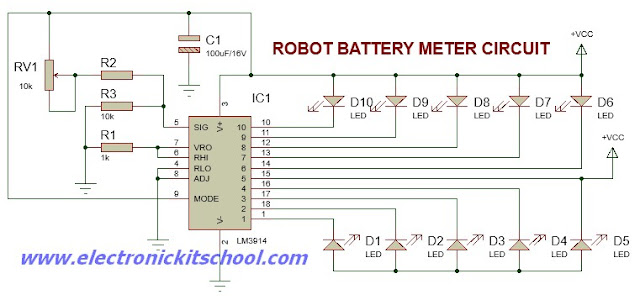## Sunday, September 4, 2016

### Robot Battery Voltage Level Indicator Circuit using LM3914 IC

Electronic Kit School. - When do you ever build a robot like fire extinguisher robot or line follower robot usually supply from small power bank with provide 4 dry cell rechargeable that arrange series to get 6V. This battery is used to supply the microcontroller IC in robot to work properly after convert to +5V.

In this article we will give you one circuit of robot battery meter or display that will be used to monitor voltage level in robot. The circuit mentioned look like in Figure 1 below. The main component to give this function is LM3914 IC.

CircuitFigure 1. Robot Battery Voltage Level Indicator Circuit using LM3914 IC

Component Parts

1. LM3914 IC
2. Resistors
3. LED
4. Potentiometer
5. Capacitor
Description

Circuit like in Figure 1 above show you about the Robot Battery Voltage Level Indicator Circuit using LM3914 IC that can produce battery voltage level indicator to indicate the voltage used in robot design. The LM3914 is a monolithic IC that senses analog voltage levels and can drives 10 LEDs with providing a linear analog display.

From Electroschematics site that In principle, circuit presented here is a 10-LED that can build from bargraph voltmeter module with full-scale deflection (FSD) of 10 Volts. Resistor R1 sets the on current of all LEDs at about 10mA. The low ends of the internal reference and divider are grounded and their top ends are joined together, so the voltmeter has a basic full-scale sensitivity of 1.2Volts.

But variable ranging is provided by the R3-R2-RV1 potential divider network at the input of the circuit (FSD = 1.2V(1+ (RV1+39) /10). Here, the value of R2 is 39K, and the maximum value of the 100K preset RV1 is 50K.

Thus when RV1 is zero, FSD is about 5.1Volt, but when RV1 is at its mechanical midpoint (50K) the FSD is near 10.1Volt. Remember to use precision resistors as R2,R3 (1%) and RV1 (multi-turn) for better display accuracy.